# Datatable Compare for Checking Rows Exist and a Condition is true for further process

I want To Compare Two dataTables And Acheck if The Data of dt1 Column1 Exist In Dt2 Column If true then i have to Check Condition Like Dt1 qty is less than dt2 Qty if this condition is true then i have use dt1 for further process
Dt1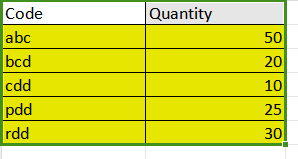Dt2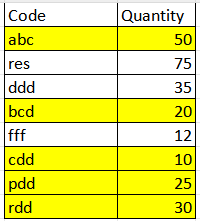Thanks

@Prateek_Gulab_Pathak

do you want to check one by one for every row or you want to check all the rows at once

try this once

for comparision use the below query

d1.aseumerable.where(function(x) dt2.asenumerable.any(function(r) cdbl(x("Quantity).tostring)<cdbl(r(“Quantity”).tostring))).copytodatatable

1 Like

thanks for the reply but i want to Check in One go if Rows Exist and quantity is less than or equal

We could try by performing a `Join Datatables` operation with Join Type as Left Join and DT1 as the First Datatable and DT2 as the Second Datatable. We should be able to get the matching data that is avaiable in DT1 and match it DT2 rows. We could then perform the check with the updated datatable by comparing the Column values of Code if there are no empty values and check Quantities if they are equal or less than Quantity from DT2.

From Debug Panel Summarisation :

Expression used to Check if the Condition Satisfies :

``````OutputDT.AsEnumerable.All(Function(x)Not String.IsNullOrWhiteSpace(x("Code_1").ToString) AndAlso CInt(x("Quantity").ToString) <= CInt(x("Quantity_1").ToString) )
``````

Here, the `OutputDT` is the resultant datatable from `Join Datatables` activity. The above Expression could be used directly in an `If` Activity.

Hi

I think u have already created a topic on this earlier and it got resolved
Would recommend not to create duplicate post

@Prateek_Gulab_Pathak

thanks @supermanPunch for reply but my data is going in the false condition again and again here is my sample data
DT1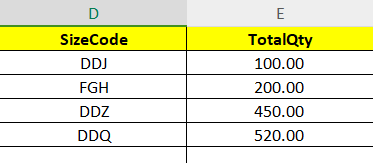dT2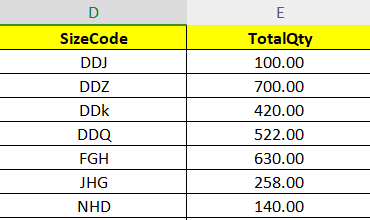I TRIED THIS CONDITION AND APPOSITE OF THIS ALSO
dt_comman.AsEnumerable.All(Function(x)Not String.IsNullOrWhiteSpace(x(“SizeCode”).ToString) AndAlso CDbl(x(“TotalQty”)) <= CDbl(x(“TotalQty_1”)) )

BUT THE SOLUTION WAS NOT CORRECT I TRIED WITH DIFFRENT DATA SET

Would it possible for you to also show case the execution in Debug Mode as I have provided ?

Would need to check the data representation/values in the Datatable.

Fine
But I’m surprised on why that topic is closed then @Prateek_Gulab_Pathak

So did we try with this join method as mentioned in ur previous post

Dt_Output = (From d1 In dt1.AsEnumerable Join d2 In dt2.AsEnumerable On d1(0) Equals d2(0) Where d1(1) < d2(1) Select d1).CopyToDataTable()

d1 and d2 as datatables of those two tables read with excel activity

Cheers @Prateek_Gulab_Pathak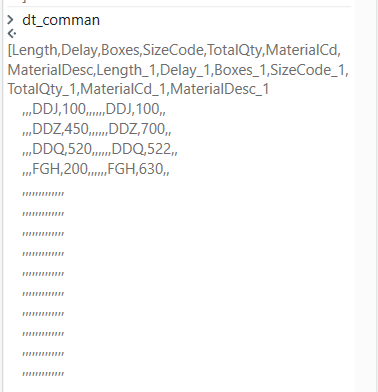yes i tried this also but it is showing it is not in correct format

Can us share the exact error message if possible
@Prateek_Gulab_Pathak

It seems that there are empty row values in your Datatables, Could you check by first performing the update of the Datatable with the below Expression :

``````OutputDT = OutputDT.AsEnumerable.Where(Function(x)Not x.ItemArray.All(Function(y)String.IsNullOrWhiteSpace(y.ToString.Trim))).CopyToDatatable
``````

You could then check the condition provided previously :

1 Like

(From row1 In dt_re11.AsEnumerable() Join row2 In dt_input.AsEnumerable() On row1(“SizeCode”).ToString Equals row2(“SizeCode”).toString Where Cint(row1(“TotalQty”).ToString) < Cint(row2(“TotalQty”).ToString) Select row1).CopyToDataTable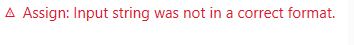1 Like

@Prateek_Gulab_Pathak

`Dt1.AsEnumerable.Where(function(x) dt2.AsEnumerable.Any(function(y) y(0).ToString.Equals(x(0).ToString) AndAlso If(x(1).ToString.Trim.IsNumeric,Cdbl(x(1).ToString.Trim),0) < If(y(1).ToString.Trim.IsNumeric,cdbl(y(1).ToString.Trim),0))).CopyToDataTable`

Cheers

Cool

We need convert the values to double instead of integer if your data contains decimal or floating-point numbers. To do that, you would replace `CInt` with `CDbl` in your LINQ expression.

Something like this

``````(From row1 In dt_re11.AsEnumerable()
Join row2 In dt_input.AsEnumerable() On row1("SizeCode").ToString Equals row2("SizeCode").ToString
Where Double.TryParse(row1("TotalQty").ToString, Nothing) AndAlso Double.TryParse(row2("TotalQty").ToString, Nothing) AndAlso
CDbl(row1("TotalQty")) < CDbl(row2("TotalQty"))
Select row1).CopyToDataTable
``````

Cheers @Prateek_Gulab_Pathak

1 Like

thanks But i tried that also same error was there

@supermanPunch now i have 0 also there in datatable as i have applied excel calculation it is showing 0 if there is now data for calculate

Could you show us the Joined Datatable in Debug Mode once again (The One not working), just to get a clear idea on what is happening with the new data ?